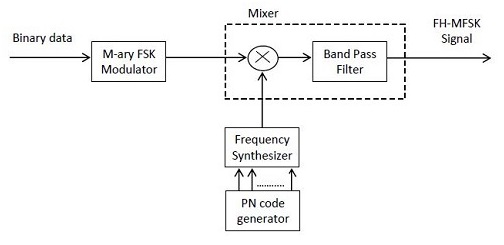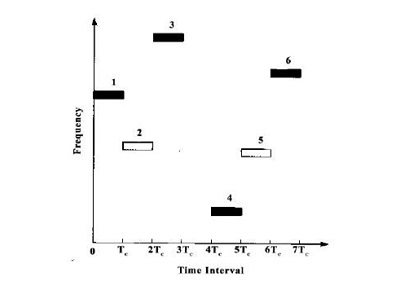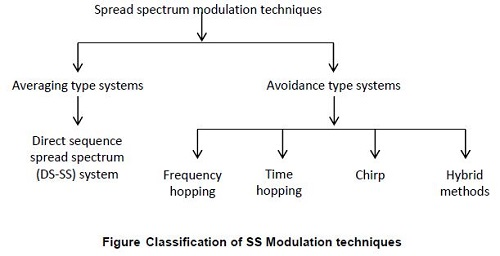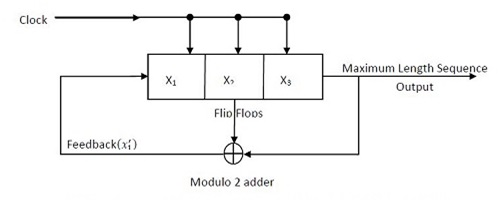Tuesday, 28 December 2021Fast Frequency Hopping Spread Spectrum Block Diagram:

Fast-hopped signals occur in the FH system when there are multiple hops per symbol. As a result, the hop rate Rh is an integer multiple of the MFSK symbol rate in fast-frequency hopping. During the transmission of a single symbol, the carrier frequency will hop or shift numerous times. As a result, each hop is a chip in a fast FH-MFSK system.

Figure 1: Fast FH-MFSK demodulator

In general, fast frequency hopping is used to overcome a smart jammer's technique, which entails two steps: measuring the spectral composition of the broadcast signal and returning the interfering signal to the same frequency range.

Before the jammer can execute these two operations, the transmitted signal must be jumped to a new carrier frequency.

Non-coherent detection is used to recover data at the receiver. The detecting process, on the other hand, differs from that utilized in a slow FH-MFSK receiver. A typical rapid FH-MFSK demodulator is seen in Figure 1.

The signal is first de-hopped using a PN generator that is identical to the one used in the transmitter. After that, a low pass filter with a bandwidth equal to the data bandwidth is used to filter the signal. A bank of 'M' envelope detectors is used to demodulate the filtered signal.

A clipping circuit and an accumulator follow each envelope detector. In the event of a deliberate jammer or other powerful unanticipated interference, the clipping circuit plays a crucial role. On a chip-by-chip basis, the demodulator does not make symbol judgments. The energy from the N chips is gathered and stored. After adding the energy from the Nth chip to the energy from the N-1 preceding ones, the demodulator chooses the symbol that corresponds to the accumulator with the most energy.

Friday, 24 December 2021We have a slow-hopped signal in the FH system when the hopping is done at the symbol rate. As a result, the symbol rate Rs of the MFSK signal is an integer multiple of the hop rate Rh when using slow-frequency hopping, indicating that many symbols are transmitted on each frequency hop.

Transmitter:

The block diagram of a slow-frequency hopping FH-MFSK transmitter is shown in Figure 1.

The incoming binary data is first sent via an M-ary FSK modulator. A Mixer receives the resultant M-ary FSK modulated signal. The Mixer is made up of a multiplier and a bandpass filter (BPF).

Figure 1: FH-MFSK Transmitter

A digital frequency synthesizer provides the other input to the mixing block. A PN code generator controls the frequency synthesizer. As a result, a carrier created by the frequency synthesizer modulates the M-ary FSK modulated signal once again. The sum and difference frequencies are produced by the Mixer in two outputs. Only the sum frequency signal, which is the FH-MFSK signal, is selected by the bandpass filter that follows the mixer. Then the signal is transmitted.

• M symbols can be transmitted using the M-ary FSK system, where M=2K. The number of bits in the input binary data that make up one symbol is denoted by k.

• Each of these M symbols will be assigned a different frequency by the M-ary FSK modulator.

• The frequency hop is the output of the synthesizer at a specific point in time.

• The PN generator's output bits vary at random. As a result, the synthesizer's output frequency will fluctuate at random.

• To create the transmitted signal, each frequency hop is combined with the MFSK signal.

• If the number of consecutive bits at the PN generator's output is n, the total number of frequency hops is 2n.

• The sum of all frequency hops determines the entire bandwidth of the transmitted FH-MFSK signal. As a result, the transmitted FH-MFSK signal has an extremely wide bandwidth, on the order of a few GHz.

A block diagram of a slow-frequency hopping FH-MFSK receiver is shown in Figure 2.

• The received signal is sent into the Mixer as an input. The digital frequency synthesizer provides the mixer with the other input.

• A PN code generator powers the frequency synthesizer. The PN code generator at the transmitter is synced with this generator.

• As a result, the frequency hops generated at the synthesizer output will be the same as those produced at the transmitter.

• The sum and difference frequencies are produced by the mixer in two outputs. Only the difference frequency, which is the MFSK signal, is selected by the bandpass filter. As a result, the frequency hopping is eliminated by the mixer.

• After then, the MFSK signal is sent into a non-coherent MFSK detector. For non-coherent MFSK detection, a bank of M non-coherent matching filters is utilized.

Each matching filter corresponds to one of the MFSK signal's tones. By selecting the greatest filter output, an approximation of the original symbol sent may be derived.

• For an FH/MFSK system,

(i) The chip rate, Rc = max (Rh, Rs) ------------------- (1)

where Rh is the hop rate and Rs is the symbol rate

(ii) The transmission of several symbols per hop characterizes a slow FH/MFSK system. As a result, in a sluggish FH/MFSK system, each symbol is referred to as a chip.

(iii) We can relate all rates as

Rc = Rs = 𝑅𝑏/𝑘 ≥ Rh ----------------- (2)

where k = log2𝑀

(iv) Processing gain, PG = Bandwidth of Spread signal / Bandwidth of unspread signal

Let fs be the symbol frequency and 2n be the number of frequency hops

Then, Processing gain, PG = 2𝑛𝑓𝑠/𝑓𝑠 = 2n -------------- (3)

(v) Probability of error, Pe = ½ 𝑒𝑟𝑏𝑅𝑐/2 ---------------- (4)

Thursday, 16 December 2021The use of a PN sequence to modulate a phase shift keyed signal allows immediate spreading of the transmission bandwidth in Direct sequence spread spectrum systems (DS-SS). A different technique that can be used is the frequency hopping spread spectrum (FH-SS) system. The transmitted signal's spectrum is spread sequentially in FH-SS by randomly hopping the data modulated carrier from one frequency to the next.

Thus, a Frequency-hopped Spread Spectrum (FH-SS) system is a type of spread spectrum in which the carrier hops from one frequency to another at random.

Basic Principle

The available channel bandwidth in an FH-SS communication system is split into a large number of contiguous frequency slots. The transmitted signal occupies one or more of the available frequency slots at each signalling period. The frequency slots in each signalling interval are chosen pseudorandomly from a PN generator's output. Figure 1 shows a specific FH pattern in the time-frequency plane.

Figure 1: Example of a Frequency - Hopped (FH) Pattern

Reason for employing M-ary FSK modulation

M-ary frequency shift keying is a popular modulation scheme for FH systems (MFSK). The combination is simply referred to as FH/MFSK. Although PSK modulation performs better than FSK in the AWGN channel, maintaining phase coherence in

Although PSK modulation performs better than FSK in the AWGN channel, maintaining phase coherence in

(i) The synthesis of frequencies employed in the hopping pattern

(ii) the signal's propagation through the channel as it hops from one frequency to another over a large bandwidth.

With FH spread spectrum transmissions, FSK modulation with non-coherent detection is typically used.

Types of Frequency hopping

We consider the rate at which the hops occur since frequency hopping does not cover the complete spread spectrum instantaneously. Frequency-hopping may be broadly classified into two types (both of which are technology-independent). They are namely:

1) Slow-frequency hopping

2) Fast-frequency hopping

Slow-frequency hopping:

We have a slow-hopped signal in the FH system if the hopping is performed at the symbol rate. As a result, with slow-frequency hopping, the MFSK signal's symbol rate Rs is an integer multiple of the hop rate Rh, implying that various symbols are transmitted per each frequency hop.

Frequency hopping example:

An example of frequency hopping is shown in Figure 2.

Figure 2: Example of Frequency Hopping

• The input binary sequence data rate is: Rb=150bits/s

• The modulation is 8-ary FSK.

• Then the symbol rate is Rs=𝑅𝑏/𝑘 = 150/log28 = 50 bits/s

• The symbol interval is Ts = 1/𝑅𝑠 = 1/50 = 20ms

• The frequency is hopped once per symbol. Thus the hopping rate is given as Rh=50hops/s.

• The abscissa (x-axis) of the figure's time-bandwidth plane represents time, while the ordinate (y-axis) denotes hopping bandwidth.

• There is an 8-ary FSK symbol-to-tone mapping available. The data band's non-fixed centre frequency is designated as f0.

• The tone separation is Δf = 1/𝑇𝑠 =1/20𝑚𝑠= 50Hz.

• At the top, there is a normal binary data sequence. The bits are grouped three at a time to generate symbols since the modulation is 8-ary FSK.

• According to symbol-to-tone assignment, a single-sideband tone (offset from f0) would be transmitted.

• f0 hops to a new position in the hopping bandwidth for each new symbol. f0+25Hz assignment is done for the first symbol in the data sequence 011. f0 is shown with a dashed line and the symbol tone f0+25Hz is shown with a solid line in the figure.

• Likewise, f0 - 125Hz assignment is done for the second symbol 110. For the third symbol 001, f0 + 125Hz assignment is done. The centre frequency f0 hops to a new position for each symbol.

Frequency hopping with diversity:

Robustness is the capacity of a sent signal to withstand channel impairments such as noise, jamming, fading, and so on in communication. A signal containing many duplicate copies, each delivered on a different frequency, has a better chance of surviving than a single signal of the same type.

Multiple broadcasts of the same signal at various frequencies that are spaced apart in time are referred to as diversity. The higher the signal's variety, the more resistant it is to random interference.

We may extend the frequency hopping example illustrated in Figure 2 to show the beneficial effect of diversity. A chip repeat factor of N=4 is used to introduce frequency hopping diversity. The effect of diversity is seen in Figure 3.

Figure 3: Frequency hopping with diversity (N =4)

• There are now four columns for each 20ms symbol interval, corresponding to the four distinct chips to be transmitted for each symbol.

• Each symbol is now transmitted four times. The centre frequency f0 is hopped to a new part of the hopping band for each transmission.

• The chip interval is Tc = 𝑇𝑠/𝑁 = 20𝑚𝑠/4 = 5ms.

• The hopping rate is Rh= 𝑅𝑏/log28 . N = 150 x 4/3 = 200 hops/s.

• To obtain orthogonality, the spacing between frequency tones must also change. Hence the tone separation is Δf = 1/𝑇𝑠 .N =4/20𝑚𝑠= 200Hz.

• Thus, the resulting transmissions provide a more robust signal than those that do not have such diversity.

Fast-frequency hopping:

If there are multiple hops per symbol in the FH system, we have a fast-hopped signal. As a result, the hop rate Rh in fast-frequency hopping is an integer multiple of the MFSK symbol rate. During the transmission of a single symbol, the carrier frequency will vary or hop numerous times. Thus, each hop in a fast FH-MFSK system is a chip.

1. The PG ( processing gain) is higher than the DS-SS system's processing gain.

2. The distance between two points does not affect synchronisation.

3. The acquisition time for a serial search system using FH-SS is reduced.

1. The FH-SS system's bandwidth is high (in GHz).

2. Digital frequency synthesisers, which are complex and expensive, are required.

Applications of FHSS system:

1) For mobile communication, CDMA systems based on FH spread spectrum signals are particularly attractive.

2) The Wi-Fi standard for wireless local area networks (WLAN).

3) The Bluetooth WPAN (Wireless Personal Area Network) standard

Fast hopping Versus Slow hopping:

The table compares the performance of fast hopping and slow hopping systems.

 SI No. Slow frequency hopping Fast frequency hopping 1. For each frequency hop, more than one symbol is transmitted. To transmit one symbol, multiple frequency hops are needed. 2. Chip rate and symbol rate are equal. The chip rate is greater than the symbol rate. 3. The symbol rate is larger than the hop rate. Hop rate is bigger than Symbol rate. 4. One or more symbols are transmitted using the same carrier frequency. In different hops, one symbol is transmitted over multiple carriers 5. If the carrier frequency in one hop is known, a jammer can detect this signal. Since one symbol is transmitted using multiple carrier frequencies, a jammer will be unable to detect it.

The following two examples can be used to compare slow and fast hopping performance:

1) Figure 4 shows the chip as part of an FH-MFSK system.

Figure 4: Chip in the context of an FM-MFSK System

• A fast frequency hopping example is shown in Figure 4 (a). The data symbol rate is 30 symbols per second, and frequency hopping is 60 hops per second. The waveform s(t) over one symbol time (1/30s) is shown in the figure. A new frequency hop is responsible for the waveform shift in (the middle of) s(t).

• A slow frequency hopping example is shown in Figure 4 (b). Although the data symbol rate remains at 30 symbols per second, the frequency hopping rate has been lowered to 10 hops per second. The waveform s(t) is shown across three symbols (1/10s).

2) The comparison for a binary FSK system is shown in Figure 5.

• For a binary FSK system, Figure (a) shows an example of rapid frequency hopping. N=4 is the diversity. Per bit, four chips are transmitted. The hop duration is the chip duration.

Figure 5: Comparison for a binary FSK system

• Figure (b) shows an example of a binary FSK system of slow frequency hopping. In this case, three bits are transferred throughout the time of a single hop. In this case, the bit duration is the chip duration.

Monday, 13 December 2021Direct Sequence Spread Spectrum Block Diagram

Direct Sequence Spread Spectrum Block Diagram

The SS modulation techniques may be divided into two groups: averaging type systems and avoidance type systems. By averaging the interference over a long period, averaging systems reduce it. The DS-SS (Direct Sequence Spread Spectrum) system is an example of an averaging system.

By making the signal avoid interference over a large fraction of time, the avoidance type systems reduce the interference. Frequency Hopping (FH) systems, Time Hopping (TH) systems, Chirp systems, and hybrid modulation systems are examples of avoidance systems.

The most significant advantage of spread spectrum modulation is that it protects against interfering signals created outside, such as jamming signals. In practice, interference suppression may be achieved using the Direct Sequence Spread Spectrum (DS-SS) approach. A bandpass channel is used for the transmission of the information signal (eg. Satellite channel). In this case, the communication system employs coherent Binary Phase Shift Keying (BPSK).

When a PN sequence is used to modulate a phase shift keyed signal in a Direct Sequence Broadened Spectrum (DS-SS) system, the transmission bandwidth is spread instantly.

DS-BPSK Transmitter

The transmitter component of the Direct Sequence Spread Spectrum with coherent BPSK is shown in the figure.  Two stages of modulation are used in the transmitter component. The NRZ encoder converts the incoming data sequence into an NRZ sequence b(t) at the first step. By applying these two sequences to the product modulator or multiplier, this sequence b(t) is utilized to modulate a broadband pseudo-noise sequence c(t). Both sequences are polar. m(t) = b(t) . c(t)  is the product sequence. The spectrum of c(t) will be identical to that of c(t). At the second step, the modulated signal m(t) is utilized to modulate the local carrier for BPSK modulation. QPSK modulation can also be used.

Hence, the second stage modulated output s(t) is a binary phase shift keyed Direct Sequence Spread (DS | BPSK) signal. Depending on the polarity of the data and PN sequences, the phase modulation ϴ(t) of the signal S(t) has one of the two values, 0 and π as shown in Table.

Table - Truth table for phase modulation ϴ(t), Radians

Waveforms

The figure illustrates the waveforms for the first stage of modulation.

Figure: Waveforms for the first stage of modulation

The waveforms for the second stage of modulation for one period of the PN sequence are shown in Figure.

Figure: Waveforms for the second stage of modulation

The receiver component of the DS-BPSK is shown in the figure.

Demodulation takes place in two stages in the receiver section. The received signal r(t) is initially subjected to coherent detection utilizing the locally produced carrier signal in the first step. This carrier signal is timed and frequency synchronized with the transmitter's carrier.

The output of the coherent detector is then de-spread in the second stage. It is multiplied by a locally produced PN sequence that is synchronized with the transmitter sequence. After despreading, the observed random signal v is integrated over a bit of time. This is utilized in decision-making since it gives an estimate of the original data sequence.

Important Observation

• In practice, the above-mentioned transmitter and receiver (DS-BPSK Transmitter and DS-BPSK Receiver) are used. Before phase modulation, spectrum spreading is conducted in the transmitter. In the receiver, phase demodulation is also done first, followed by despreading; however, the sequence of these two processes is reversed in the DS spread spectrum BPSK system model used for analysis.

• BPSK is performed first in the transmitter, followed by spectrum spreading. Similarly, at the receiver, spectrum despreading is performed first, followed by phase demodulation.

• Since spectrum spreading and BPSK are both linear processes, this is possible.

1. This system is the most effective at detecting and preventing deliberate interference (jamming).

2. For multipath signals, this system has a very high level of discrimination. As a result, the multipath interference is successfully reduced.

3. When compared to other systems, the DS-SS system outperforms them in the presence of noise.

1. The output rate of the PN code generator must be high. The length of such a series must be sufficient to ensure that it is genuinely random.

2. The acquisition time using the serial search method is too long. As a result, the DS-SS system is sluggish.

3. The varying distance between the transmitter and receiver affects synchronization.

4. The DS-SS signal is ineffective in the case of broadband interference.

Applications of DS-SS system

1. Anti-jamming application — protecting a jamming signal.

2. Signal transmission with low detectability - the signal is intentionally delivered at a very low power level. As a result, the signal is known as an LPI signal since it has a low probability of being intercepted (LPI).

3. Supporting numerous simultaneous signal transmissions on the same channel, such as with Code Division Multiple Access (CDMA) or spread spectrum multiple access (SSMA).

PERFORMANCE PARAMETERS OF DS-SS SYSTEM

A direct sequence spread spectrum system's important performance characteristics are 1) processing gain, 2) probability of error, and 3) jamming margin.

1) Processing Gain

The gain achieved by processing a spread spectrum signal over an unspread signal is referred to as the processing gain of a DS-SS system. It's also known as the spread spectrum signal's bandwidth divided by the unspread signal's bandwidth.

Thus,

Processing Gain (PG) = 𝐵𝑎𝑛𝑑𝑤𝑖𝑑𝑡ℎ 𝑜𝑓 𝑠𝑝𝑟𝑒𝑎𝑑 𝑠𝑖𝑔𝑛𝑎𝑙/𝐵𝑎𝑛𝑑𝑤𝑖𝑑𝑡ℎ 𝑜𝑓 𝑢𝑛𝑠𝑝𝑟𝑒𝑎𝑑 𝑠𝑖𝑔𝑛𝑎𝑙

The bandwidth of an unspread signal is determined by the bit rate of binary data entering the transmitter input. It is given by

Rb = 1/𝑇𝑏 ------------------- (1)

The chip rate of the PN sequence also refers to the spread spectrum signal's bandwidth. It is given by

Rc = 1/𝑇𝑐 ------------------ (2)

Therefore, Processing gain is given by

PG = 𝑅𝑐/𝑅𝑏 = (1/𝑇𝑐)/(1/𝑇𝑏) = 𝑇𝑏/𝑇𝑐

=> PG = 𝑇𝑏/𝑇𝑐 ----------------------- (3)

Also  Tb = NTc. This can be rewritten as

N = 𝑇𝑏/𝑇𝑐 ------------------- (4)

N is the spread factor, which is the number of chips per information bit.

Both PG and N are equal.  Hence

PG = N = 𝑇𝑏/𝑇𝑐 ------------------- (5)

Since it represents the advantage gained over the jammer by increasing the bandwidth of the transmitted signal, the Processing Gain (PG) is also known as the bandwidth expansion factor (Be).

2) Probability of Error

• The probability of error Pe for a coherent BPSK system can be determined as:

Where Eb is the energy per bit and 𝑁𝑜/2 is the power spectral density of white noise.

• The interference in a DS-SS BPSK system can be expressed as a wideband noise signal with a power spectral density of No/2. For the spread signal, we may write No as

No = JTc ------------------------ (7)

where J refers to the average interference power and Tc refers to chip duration or interval.

• On substituting the value of No in equation (6), the probability of error for the DS-SS-BPSK system can be calculated as:

3) Jamming Margin (Antijam characteristics)

• The bit energy to noise density ratio is represented as Eb/No. We may write No as JTC (No = JTc) for the DS-SS-BPSK system. The bit energy Eb is given by

Eb = PsTb -------------------------- (9)

Tb is the bit duration or interval, while Ps is the average signal power.

Thus  𝐸𝑏/𝑁𝑜 can be written as

𝐸𝑏/𝑁𝑜 = 𝑃𝑠𝑇𝑏/𝐽𝑇𝑐 = (𝑃𝑠/𝐽) (𝑇𝑏/𝑇𝑐) ------------------- (10)

𝐽/𝑃𝑠 = 𝑇𝑏/𝑇𝑐/𝐸𝑏/𝑁𝑜 = (𝑃𝐺/𝐸𝑏/𝑁𝑜) ------------- (11)

Since we know that PG=𝑇𝑏/𝑇𝑐

The jamming margin is defined as the ratio J/Ps. Thus, the jamming margin is the ratio of average interference power J to average signal power Ps.

If the jamming margin and processing gain are both expressed in dB (decibels), the equation for the jamming margin is:

(Jamming margin)dB = (Processing gain)dB - 10log10(𝐸𝑏/𝑁𝑜)𝑚𝑖𝑛 ----------------- (12)

where (Eb/No)min is the bit energy-to-noise density ratio required to support a given average probability of error.

Tuesday, 7 December 2021